• Buckinghamshire Tutors

# 11+ Course in a Blog: Maths, Verbal Reasoning, Essential Vocabulary - Week 7Welcome to week 7. I am so pleased to hear about the growing number of people following this blog. I am writing it because there was no such resource when I tutored my son for his 11+. I found I needed more than the books that were available, but many of the links on the Khan academy took me up to GCSE, etc so I am happy to find myself, years later sharing the links I have found most suitable. I have watched all of the videos in this blog to ensure their suitability for the 11+ student.

## Fractions

First of all proper fractions: they are less than 1, or put another way, they are parts of a whole thing but not the whole thing. You would know if you were not getting the whole pizza but only 1/3 of it – right?Anything can be split into fractions, even the floor as in the bamzooki clip below. (Beware - it is LOUD.)Click on the picture above and open the hyperlink. Or use the link below.

http://www.bbc.co.uk/bitesize/ks2/maths/number/fractions_basic/play/Click on the picture above and open the hyperlink. Or use the link below.

http://www.free-training-tutorial.com/identifying-fractions-games.html

Practise on IXL. You get ten questions a day for free, so there is no need to subscribe. I suggest you do a different section each time.

https://uk.ixl.com/math/year-5/fractions-review

S3

https://uk.ixl.com/math/year-5/fractions-of-a-whole-word-problems

## Equivalent fractions

Equivalent means equal. Equivalent fractions may look different, but they are equal. So long as we multiply the top and the bottom by the same number, they remain equal.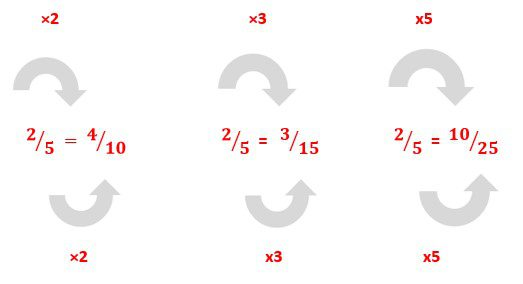When we simplify, we divide the numerator and the denominator by the same number and they remain equal.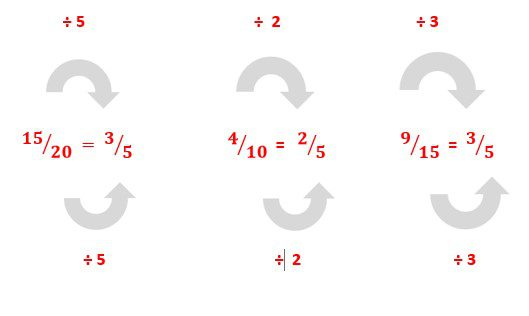Click on the picture above and open the hyperlink. Or use the link below.

http://www.free-training-tutorial.com/equivalent-fractions-games.html

Practice Equivalent Fractions

https://uk.ixl.com/math/year-5/equivalent-fractions

https://uk.ixl.com/math/year-5/find-equivalent-fractions-using-area-modelshttp://www.free-training-tutorial.com/comparing-fractions-games.html

Pratcice Ordering Fractions

https://uk.ixl.com/math/year-5/order-fractions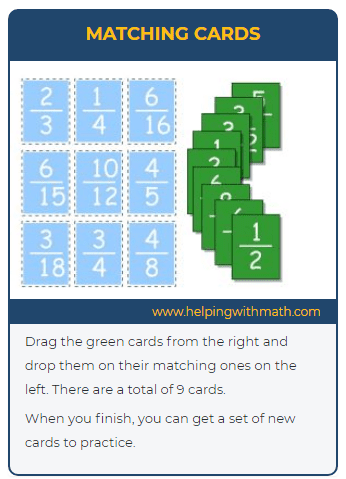http://www.free-training-tutorial.com/math-games/fractions-equivalent-matching.html

Practice simplifying Fractions

https://uk.ixl.com/math/year-5/write-fractions-in-lowest-termsCan you see that the improper fraction and the mixed fraction are equivalent? Sometimes mixed numbers are more useful, sometimes improper fractions are more useful, which you will see as you go on to become a better mathematician in the sections next week.

It is easy if they have the same denominator (number on the bottom).

Complete all sections on the menu## Adding and subtracting fractions with unlike denominators

#### There is one more step involved if they do not have the same denominator like in the example below.Find the lowest common multiple of the denominators:

This is called the ‘lowest common denominator’

We need a 6on the bottom of both of these fractions

We need to turn the thirds into sixths, but how?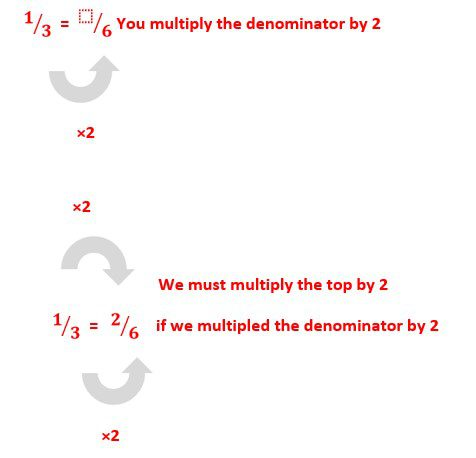You have created equivalent fractions, so now you can add them together!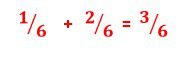Another exampleFind the lowest common multiple of the denominator:12

We need a 12 on the bottom of both of these fractions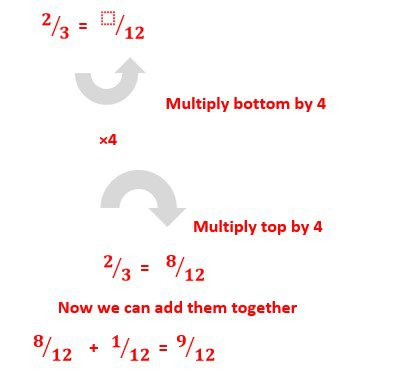Watch the video below for more examples, including when we need to change both bottom numbers, as in the picture below.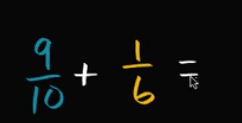## Find Fractions of a Number

This is different from adding and subtracting fractions.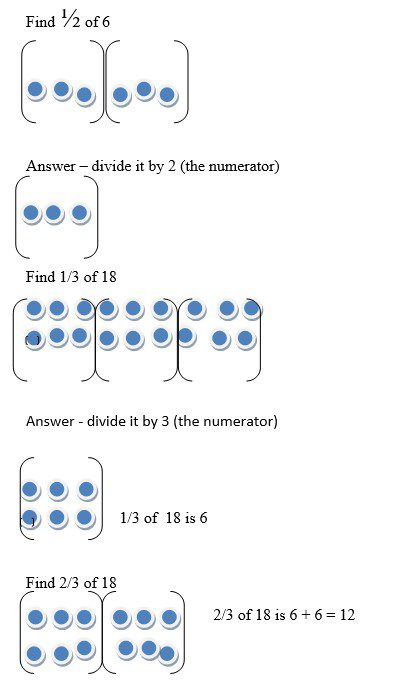Practice finding fractions of a number

S14 (Year 5)

https://uk.ixl.com/math/year-5/fractions-of-a-number

S15

https://uk.ixl.com/math/year-5/fractions-of-a-number-word-problems

If you still don’t get it, take a look at all the pages on the menu at BBC Bitesize Fractions.And, if you still don’t get, it try this site below.

https://www.mathsisfun.com/fractions.html

## Find the Missing Word

Insert the missing three-letter-word into the incomplete word.

The cat’s HRING was superhuman.

Use the meaning of the sentence to help you find the word; the word is hearing, so the missing word is EAR. H EAR ING

Here’s another example:

The COMER was broken.

What could we describe as broken? Probably a thing. The word is COMPUTER, so the missing word is PUT. COM PUT ER

You try this one:
1) We were tired of WAIG.

2) The WS fell off the car.

3) My dog loves his BET.

4) The boy asked if he could be EXCED.

5) She read using a CLE.

6) The girl was ENTD by the delicous smell.

7) Keep things tidy in your FER.

8) Marmite is HORLE.

9) Marmite is FASTIC.

10) The WHER was fine.

11) Everybody wants to be a CHION.

12) They work in the LABOORY.

13) He is an OUTEOUS cheat.

14) It was dark in the narrow PASE.

15) PHOTOGHY is a great hobby.

16) The storyline was GPING.

17) Dying young is a TRDY.

18) WHVER you do, do it well.

20) I shouted until I was HSE.

Answers to Find the Missing Word

### VocabularyNext week: Verbal Reasoning, Multiplying and Dividing Fractions, Vocabulary.